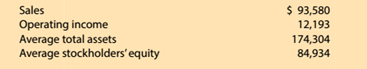Chapter 15, Problem 15.1.2MBA

Chapter
Section
Textbook Problem

Financial leverageMicrosoCortrepotied (MSFT) reported the following data (in millions) for a tern yearCompute the return on stockholders’ equity. Round to one decimal place.

To determine

Concept Introduction:

Return on Equity:

Return on Equity is the rate of return earned by the Stockholders on their investment in the company. It is calculated with the help of following formula:

Return on Equity = Net IncomeAverage Stockholders Equity

The Average stock holder's equity calculated with the help of following formula:

Average stock holders equity=( Beginning stock holders equity + Ending stock holders equity)2

To Calculate:

The Return on Stockholder's equity

Explanation

The Return on Stockholder's equity is calculated as follows:

 $in Millions Operating Income (A)$ 1...

Still sussing out bartleby?

Check out a sample textbook solution.

See a sample solution

The Solution to Your Study Problems

Bartleby provides explanations to thousands of textbook problems written by our experts, many with advanced degrees!

Get Started

(Appendix 6. 1) What is the purpose of a petty cash system?

Intermediate Accounting: Reporting And Analysis

In what ways is economics a science?

Essentials of Economics (MindTap Course List)

DuPONT ANALYSIS Doublewide Dealers has an ROA of 10%, a 2% profit margin, and an ROE of 15%. What is its total ...

Fundamentals of Financial Management, Concise Edition (with Thomson ONE - Business School Edition, 1 term (6 months) Printed Access Card) (MindTap Course List)